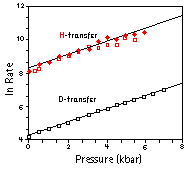Pressure Dependence of Reaction RateThe figure shows the pressure dependence of the H (top) and D (bottom) transfer rate k at room temperature. At all temperatures k follows ln k = S(T) · p with

S(1.4 K)  = 0.244 kbar-1
S(77 K)   = 0.248 kbar-1
S(150 K) = 0.317 kbar-1
S(300 K) = 0.372 kbar-1

The data at 1.4 K render a constant low temperature reaction rate k0 = 1.25 s-1 at ambient pressure .

Home Back